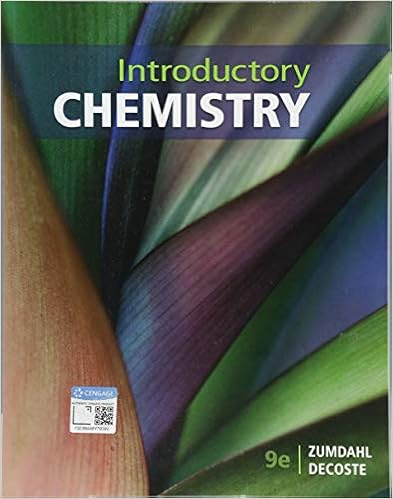# B 56 ml 2 c 60104 ton 5 d 2900 g 4 e 402 gcm 3 3 f

• Homework Help
• feicheng916
• 3
• 86% (7) 6 out of 7 people found this document helpful

This preview shows page 2 - 3 out of 3 pages.

##### We have textbook solutions for you!
The document you are viewing contains questions related to this textbook.The document you are viewing contains questions related to this textbook.
Chapter 2 / Exercise 54
Introductory Chemistry
Zumdahl/DecosteExpert Verified
b.56 mL 2
5d.2900 g e.40.2 g/cm3 43
1
5.Express the results in the proper number of significant figures. (12 points)Incorrectcheck sig. figs. for each calculation-12a.5.6792 m + 0.6 m + 4.33 m =10.6092mb.3.70 g – 2.9133 g =0.7867gc.4.51 cm × 3.6666 cm =16.536366cm^2d.(3 × 104g+ 6.827g)/(0.043 cm3– 0.021 cm3) =1.364*10^6 g/cm^3(Reference: Chang 1.35)6.Carry out the following conversions: (16 points)a.22.6 m to dm 226dmb.25.4 mg to kilograms 2.54x10^-5 kgc.556 mL to liters 0.556Ld.10.6 kg/m3to g/cm3 1.06x10^-2 g/cm3(Reference: Chang 1.39)7.The average speed of helium at 25°C is 1255 m/s. Convert this speed to miles per hour (mph). (4 points)sig. fig. error
8.How many minutes does it take light from the sun to reach Earth? (The distance
##### We have textbook solutions for you!
The document you are viewing contains questions related to this textbook.The document you are viewing contains questions related to this textbook.
Chapter 2 / Exercise 54
Introductory Chemistry
Zumdahl/DecosteExpert Verified
9.Normal body temperature is 78.0°F. Calculate this temperature in degrees Celsius and Kelvin. (4 points)
•••